# spectralDecrease

Spectral decrease for audio signals and auditory spectrograms

## Syntax

``decrease = spectralDecrease(x,f)``
``decrease = spectralDecrease(x,f,Name,Value)``

## Description

example

````decrease = spectralDecrease(x,f)` returns the spectral decrease of the signal, `x`, over time. How the function interprets `x` depends on the shape of `f`.```

example

````decrease = spectralDecrease(x,f,Name,Value)` specifies options using one or more `Name,Value` pair arguments.```

## Examples

collapse all

Read in an audio file, calculate the decrease using default parameters, and then plot the results.

```[audioIn,fs] = audioread('Counting-16-44p1-mono-15secs.wav'); decrease = spectralDecrease(audioIn,fs); t = linspace(0,size(audioIn,1)/fs,size(decrease,1)); plot(t,decrease) xlabel('Time (s)') ylabel('Decrease')```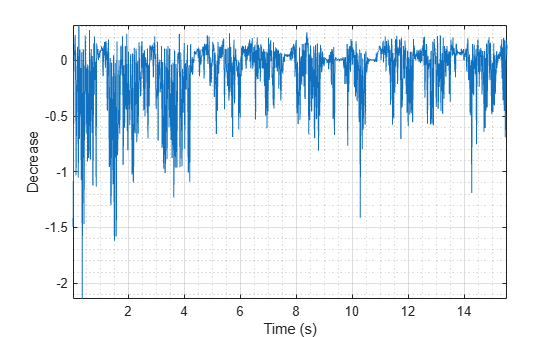Read in an audio file and then calculate the mel spectrogram using the `melSpectrogram` function.

```[audioIn,fs] = audioread('Counting-16-44p1-mono-15secs.wav'); [s,cf] = melSpectrogram(audioIn,fs);```

Calculate the decrease of the mel spectrogram over time. Plot the results.

```decrease = spectralDecrease(s,cf); t = linspace(0,size(audioIn,1)/fs,size(decrease,1)); plot(t,decrease) xlabel('Time (s)') ylabel('Decrease')```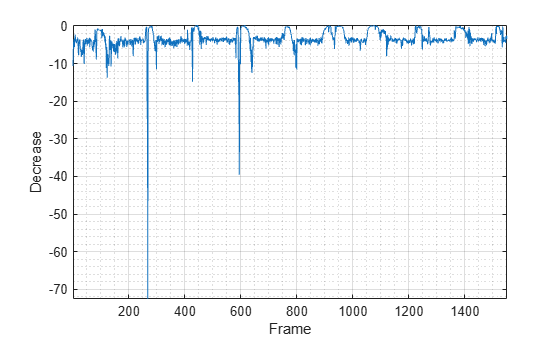`[audioIn,fs] = audioread('Counting-16-44p1-mono-15secs.wav');`

Calculate the decrease of the magnitude spectrum over time. Calculate the decrease for 50 ms Hamming windows of data with 25 ms overlap. Use the range from 62.5 Hz to `fs`/2 for the decrease calculation. Plot the results.

```decrease = spectralDecrease(audioIn,fs, ... 'Window',hamming(round(0.05*fs)), ... 'OverlapLength',round(0.025*fs), ... 'Range',[62.5,fs/2]); t = linspace(0,size(audioIn,1)/fs,size(decrease,1)); plot(t,decrease) xlabel('Time (s)') ylabel('Decrease')```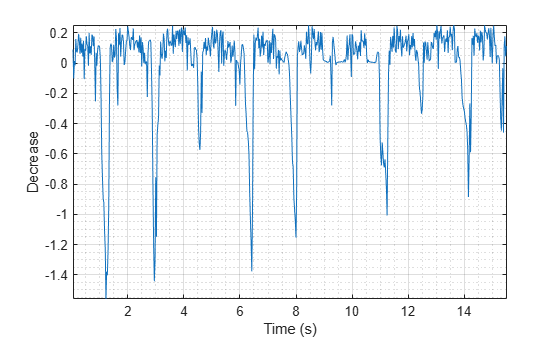Create a `dsp.AudioFileReader` object to read in audio data frame-by-frame. Create a `dsp.SignalSink` to log the spectral decrease calculation.

```fileReader = dsp.AudioFileReader('Counting-16-44p1-mono-15secs.wav'); logger = dsp.SignalSink;```

In an audio stream loop:

1. Read in a frame of audio data.

2. Calculate the spectral decrease for the frame of audio.

3. Log the spectral decrease for later plotting.

To calculate the spectral decrease for only a given input frame, specify a window with the same number of samples as the input, and set the overlap length to zero. Plot the logged data.

```while ~isDone(fileReader) audioIn = fileReader(); decrease = spectralDecrease(audioIn,fileReader.SampleRate, ... 'Window',hamming(size(audioIn,1)), ... 'OverlapLength',0); logger(decrease) end plot(logger.Buffer) ylabel('Decrease')```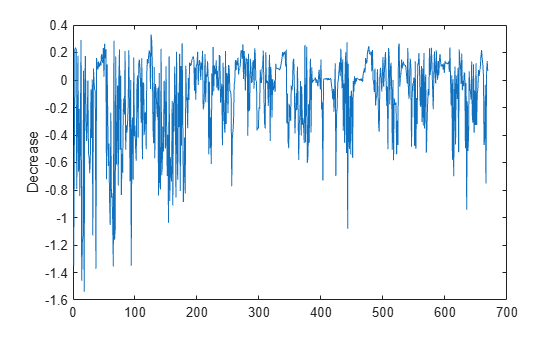Use `dsp.AsyncBuffer` if

• The input to your audio stream loop has a variable samples-per-frame.

• The input to your audio stream loop has an inconsistent samples-per-frame with the analysis window of `spectralDecrease`.

• You want to calculate the spectral decrease for overlapped data.

Create a `dsp.AsyncBuffer` object, reset the logger, and release the file reader.

```buff = dsp.AsyncBuffer; reset(logger) release(fileReader)```

Specify that the spectral decrease is calculated for 50 ms frames with a 25 ms overlap.

```fs = fileReader.SampleRate; samplesPerFrame = round(fs*0.05); samplesOverlap = round(fs*0.025); samplesPerHop = samplesPerFrame - samplesOverlap; win = hamming(samplesPerFrame); while ~isDone(fileReader) audioIn = fileReader(); write(buff,audioIn); while buff.NumUnreadSamples >= samplesPerHop audioBuffered = read(buff,samplesPerFrame,samplesOverlap); decrease = spectralDecrease(audioBuffered,fs, ... 'Window',win, ... 'OverlapLength',0); logger(decrease) end end release(fileReader)```

Plot the logged data.

```plot(logger.Buffer) ylabel('Decrease')```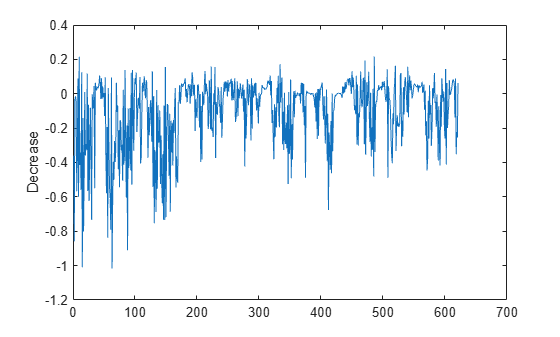## Input Arguments

collapse all

Input signal, specified as a vector, matrix, or 3-D array. How the function interprets `x` depends on the shape of `f`.

Data Types: `single` | `double`

Sample rate or frequency vector in Hz, specified as a scalar or vector, respectively. How the function interprets `x` depends on the shape of `f`:

• If `f` is a scalar, `x` is interpreted as a time-domain signal, and `f` is interpreted as the sample rate. In this case, `x` must be a real vector or matrix. If `x` is specified as a matrix, the columns are interpreted as individual channels.

• If `f` is a vector, `x` is interpreted as a frequency-domain signal, and `f` is interpreted as the frequencies, in Hz, corresponding to the rows of `x`. In this case, `x` must be a real L-by-M-by-N array, where L is the number of spectral values at given frequencies of `f`, M is the number of individual spectra, and N is the number of channels.

• The number of rows of `x`, L, must be equal to the number of elements of `f`.

Data Types: `single` | `double`

### Name-Value Arguments

Specify optional comma-separated pairs of `Name,Value` arguments. `Name` is the argument name and `Value` is the corresponding value. `Name` must appear inside quotes. You can specify several name and value pair arguments in any order as `Name1,Value1,...,NameN,ValueN`.

Example: `'Window',hamming(256)`

Note

The following name-value pair arguments apply if `x` is a time-domain signal. If `x` is a frequency-domain signal, name-value pair arguments are ignored.

Window applied in the time domain, specified as the comma-separated pair consisting of `'Window'` and a real vector. The number of elements in the vector must be in the range [1, `size(x,1)`]. The number of elements in the vector must also be greater than `OverlapLength`.

Data Types: `single` | `double`

Number of samples overlapped between adjacent windows, specified as the comma-separated pair consisting of `'OverlapLength'` and an integer in the range [0, `size(Window,1)`).

Data Types: `single` | `double`

Number of bins used to calculate the DFT of windowed input samples, specified as the comma-separated pair consisting of `'FFTLength'` and a positive scalar integer. If unspecified, `FFTLength` defaults to the number of elements in the `Window`.

Data Types: `single` | `double`

Frequency range in Hz, specified as the comma-separated pair consisting of `'Range'` and a two-element row vector of increasing real values in the range [0, `f`/2].

Data Types: `single` | `double`

Spectrum type, specified as the comma-separated pair consisting of `'SpectrumType'` and `'power'` or `'magnitude'`:

• `'power'` –– The spectral decrease is calculated for the one-sided power spectrum.

• `'magnitude'` –– The spectral decrease is calculated for the one-sided magnitude spectrum.

Data Types: `char` | `string`

## Output Arguments

collapse all

Spectral decrease in Hz, returned as a scalar, vector, or matrix. Each row of `decrease` corresponds to the spectral centroid of a window of `x`. Each column of `decrease` corresponds to an independent channel.

## Algorithms

The spectral decrease is calculated as described in :

`$\text{decrease}=\frac{\sum _{k={b}_{1}+1}^{{b}_{2}}\frac{{s}_{k}-{s}_{{b}_{1}}}{k-1}}{\sum _{k={b}_{1}+1}^{{b}_{2}}{s}_{k}}$`

where

• sk is the spectral value at bin k.

• b1 and b2 are the band edges, in bins, over which to calculate the spectral decrease.

 Peeters, G. "A Large Set of Audio Features for Sound Description (Similarity and Classification) in the CUIDADO Project." Technical Report; IRCAM: Paris, France, 2004.

## Support평가판 신청